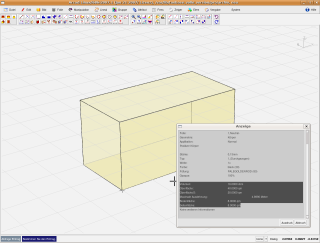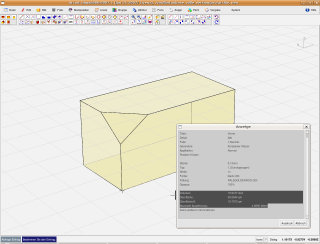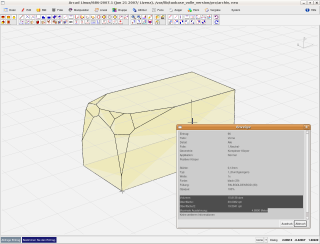#### Dimensions, easy to calculate?

If a calculation is based on a simple geometrical basis, a mass can also always be calculated after basic rules.
But how does it look with a surface which allows absolutely no simple calculation?
Or how do you calculate the volume and the surface of a stone found in the nature?
ARCAD can exactly calculate any surfaces and volumes up to the smallest decimal place.
In the architecture such calculations often appear.
Calculate only the earth excavation or the excavation of a building pit in the foothills.

#### Calculation of areas

The placing of the calculation of areas is the assignment of space-determining parametres to free areas.
These areas serve the calculation of the living space and floor space of a project.
Besides, the areas are sampled in comprehensible single surfaces which are shown in the calculation of areas.

Length
Calculation of the length of an entry.

Surface
This function calculates the square metres of a surface.

Volume
Calculates the volume of a solid.

Volume of a surface
Calculates the volume of a surface.

Surface of the solid
Calculate the surface of a complicated solid.

Surface of the solid / 2
Calculates the surface of a complicated solid and divides the result by 2.
This function can calculate the surface of big structual slabs which are shown as a solid.

Item (element)
An entry is provided with the number 1.
Calculates from an assignment entry the amount 1 with the quantity unit item.
Operates only with elements.

Wall ground surface
Calculates the ground surface of a wall opening or a wall item.

Wall volume
Calculates the wall surface or the wall volume less the apertures or doorways.
In order to assign these attributes, you must select the wall and the openings.

Wall length
Calculates the length of a wall.

Wall length internal
Calculates the internal length of a wall.
The internal length is the length of the wall in running direction to the left.

Wall length external
Calculates the external length of a wall.
The external length is the length of the wall in running direction to the right.

Wall length internal and external
Calculates the external length of a wall.
The external length is the length of the wall in running direction to the left and to the right.

Wall area
Calculates the wall area.

Wall area internal
Calculates the inner surface of a wall.
The inner surface is the area of the wall in running direction to the left.

Wall area external
Calculates the external surface of a wall.
The external surface is the area of the wall in running direction to the right.

Wall area internal and external
Calculates the external and internal wall area.
The external surface is the wall area in running direction to the left and to the right.

The maximum length
Calculates the maximum expansion of a solid.

The maximum surface
Calculates the maximum surface of a solid.

#### A block with 4x2x2 metresWe create a simple block with the edge length of 4x2x2 metres.
ARCAD correctly calculates a volume of 16 cubic metres.
Because this block has a simple geometrical form, the floorspace and upper surface will be displayed, too.
Besides, we receive the whole wrapping surface.

#### A little bit truncatedWe have now truncated from this block a piece.
Try to manually calculate the correct volume of this newly formed block.
You will find out that this becomes already much more difficult for you.
ARCAD also calculates in this case the correct volume and surface.
You can click on the image with the mouse to enlarge it.

#### Almost a hand axThe shown object should only offer one little example.
ARCAD also processes because of the arithmetic strength big and very large objects.
Coherent and closed objects are calculated and sorted according to the attributes.
The arithmetic results are comprehensible and are printed out in a list (report).
Continuing programs can read in these data with an interface and analyze.